# 9.7: Use Properties of Rectangles, Triangles, and Trapezoids (Part 2)

•• OpenStax
• OpenStax
$$\newcommand{\vecs}{\overset { \rightharpoonup} {\mathbf{#1}} }$$ $$\newcommand{\vecd}{\overset{-\!-\!\rightharpoonup}{\vphantom{a}\smash {#1}}}$$$$\newcommand{\id}{\mathrm{id}}$$ $$\newcommand{\Span}{\mathrm{span}}$$ $$\newcommand{\kernel}{\mathrm{null}\,}$$ $$\newcommand{\range}{\mathrm{range}\,}$$ $$\newcommand{\RealPart}{\mathrm{Re}}$$ $$\newcommand{\ImaginaryPart}{\mathrm{Im}}$$ $$\newcommand{\Argument}{\mathrm{Arg}}$$ $$\newcommand{\norm}{\| #1 \|}$$ $$\newcommand{\inner}{\langle #1, #2 \rangle}$$ $$\newcommand{\Span}{\mathrm{span}}$$ $$\newcommand{\id}{\mathrm{id}}$$ $$\newcommand{\Span}{\mathrm{span}}$$ $$\newcommand{\kernel}{\mathrm{null}\,}$$ $$\newcommand{\range}{\mathrm{range}\,}$$ $$\newcommand{\RealPart}{\mathrm{Re}}$$ $$\newcommand{\ImaginaryPart}{\mathrm{Im}}$$ $$\newcommand{\Argument}{\mathrm{Arg}}$$ $$\newcommand{\norm}{\| #1 \|}$$ $$\newcommand{\inner}{\langle #1, #2 \rangle}$$ $$\newcommand{\Span}{\mathrm{span}}$$$$\newcommand{\AA}{\unicode[.8,0]{x212B}}$$

## Use the Properties of Triangles

We now know how to find the area of a rectangle. We can use this fact to help us visualize the formula for the area of a triangle. In the rectangle in Figure $$\PageIndex{9}$$, we’ve labeled the length b and the width h, so it’s area is bh.Figure $$\PageIndex{9}$$ - The area of a rectangle is the base, b, times the height, h.

We can divide this rectangle into two congruent triangles (Figure $$\PageIndex{10}$$). Triangles that are congruent have identical side lengths and angles, and so their areas are equal. The area of each triangle is one-half the area of the rectangle, or $$\dfrac{1}{2}$$bh. This example helps us see why the formula for the area of a triangle is A = $$\dfrac{1}{2}$$bh.Figure $$\PageIndex{10}$$ - A rectangle can be divided into two triangles of equal area. The area of each triangle is one-half the area of the rectangle.

The formula for the area of a triangle is A = $$\dfrac{1}{2}$$bh, where b is the base and h is the height. To find the area of the triangle, you need to know its base and height. The base is the length of one side of the triangle, usually the side at the bottom. The height is the length of the line that connects the base to the opposite vertex, and makes a 90° angle with the base. Figure $$\PageIndex{11}$$ shows three triangles with the base and height of each marked.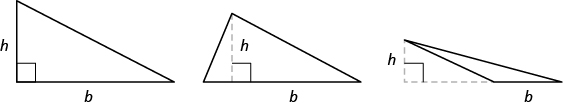Figure $$\PageIndex{11}$$ - The height h of a triangle is the length of a line segment that connects the the base to the opposite vertex and makes a 90° angle with the base.

##### Definition: Triangle Properties

For any triangle ΔABC, the sum of the measures of the angles is 180°.$m \angle A + m \angle B + m \angle C = 180°The perimeter of a triangle is the sum of the lengths of the sides.P = a + b + cThe area of a triangle is one-half the base, b, times the height, h.A = \dfrac{1}{2} bh$##### Example $$\PageIndex{9}$$:

Find the area of a triangle whose base is 11 inches and whose height is 8 inches.

Solution

 Step 1. Read the problem. Draw the figure and label it with the given information.Step 2. Identify what you are looking for. the area of the triangle Step 3. Name. Choose a variable to represent it. let A = area of the triangle Step 4.Translate. Write the appropriate formula. Substitute.Step 5. Solve the equation. A = 44 square inches Step 6. Check. $$\begin{split} A &= \dfrac{1}{2} bh \\ 44 &\stackrel{?}{=} \dfrac{1}{2} (11)8 \\ 44 &= 44\; \checkmark \end{split}$$ Step 7. Answer the question. The area is 44 square inches.
##### Exercise $$\PageIndex{17}$$:

Find the area of a triangle with base 13 inches and height 2 inches.

13 sq. in.

##### Exercise $$\PageIndex{18}$$:

Find the area of a triangle with base 14 inches and height 7 inches.

49 sq. in.

##### Example $$\PageIndex{10}$$:

The perimeter of a triangular garden is 24 feet. The lengths of two sides are 4 feet and 9 feet. How long is the third side?

Solution

 Step 1. Read the problem. Draw the figure and label it with the given information.Step 2. Identify what you are looking for. length of the third side of a triangle Step 3. Name. Choose a variable to represent it. Let c = the third side Step 4.Translate. Write the appropriate formula. Substitute in the given information.Step 5. Solve the equation. $$\begin{split} 24 &= 13 + c \\ 11 &= c \end{split}$$ Step 6. Check. $$\begin{split} P &= a + b + c \\ 24 &\stackrel{?}{=} 4 + 9 + 11 \\ 24 &= 24\; \checkmark \end{split}$$ Step 7. Answer the question. The third side is 11 feet long.
##### Exercise $$\PageIndex{19}$$:

The perimeter of a triangular garden is 48 feet. The lengths of two sides are 18 feet and 22 feet. How long is the third side?

8 ft

##### Exercise $$\PageIndex{20}$$:

The lengths of two sides of a triangular window are 7 feet and 5 feet. The perimeter is 18 feet. How long is the third side?

6 ft

##### Example $$\PageIndex{11}$$:

The area of a triangular church window is 90 square meters. The base of the window is 15 meters. What is the window’s height?

Solution

 Step 1. Read the problem. Draw the figure and label it with the given information.Step 2. Identify what you are looking for. height of a triangle Step 3. Name. Choose a variable to represent it. Let h = the height Step 4.Translate. Write the appropriate formula. Substitute in the given information.Step 5. Solve the equation. $$\begin{split} 90 &= \dfrac{15}{2} h \\ 12 &= h \end{split}$$ Step 6. Check. $$\begin{split} A &= \dfrac{1}{2} bh \\ 90 &\stackrel{?}{=} \dfrac{1}{2} \cdot 15 \cdot 12 \\ 90 &= 90\; \checkmark \end{split}$$ Step 7. Answer the question. The height of the triangle is 12 meters.
##### Exercise $$\PageIndex{21}$$:

The area of a triangular painting is 126 square inches. The base is 18 inches. What is the height?

14 in.

##### Exercise $$\PageIndex{22}$$:

A triangular tent door has an area of 15 square feet. The height is 5 feet. What is the base?

6 ft

### Isosceles and Equilateral Triangles

Besides the right triangle, some other triangles have special names. A triangle with two sides of equal length is called an isosceles triangle. A triangle that has three sides of equal length is called an equilateral triangle. Figure $$\PageIndex{12}$$ shows both types of triangles.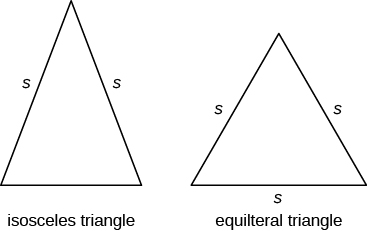Figure $$\PageIndex{12}$$ - In an isosceles triangle, two sides have the same length, and the third side is the base. In an equilateral triangle, all three sides have the same length.

##### Definition: Isosceles and Equilateral Triangles

An isosceles triangle has two sides the same length.

An equilateral triangle has three sides of equal length.

##### Example $$\PageIndex{12}$$:

The perimeter of an equilateral triangle is 93 inches. Find the length of each side.

Solution

 Step 1. Read the problem. Draw the figure and label it with the given information.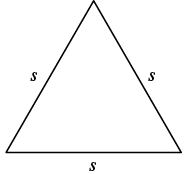Perimeter = 93 in. Step 2. Identify what you are looking for. length of the sides of an equilateral triangle Step 3. Name. Choose a variable to represent it. Let s = length of each side Step 4.Translate. Write the appropriate formula. Substitute.Step 5. Solve the equation. $$\begin{split} 93 &= 3s \\ 31 &= s \end{split}$$ Step 6. Check.$$\begin{split} 93 &= 31 + 31 + 31 \\ 93 &= 93\; \checkmark \end{split}$$ Step 7. Answer the question. Each side is 31 inches.
##### Exercise $$\PageIndex{23}$$:

Find the length of each side of an equilateral triangle with perimeter 39 inches.

13 in.

##### Exercise $$\PageIndex{24}$$:

Find the length of each side of an equilateral triangle with perimeter 51 centimeters.

17 cm

##### Example $$\PageIndex{13}$$:

Arianna has 156 inches of beading to use as trim around a scarf. The scarf will be an isosceles triangle with a base of 60 inches. How long can she make the two equal sides?

Solution

 Step 1. Read the problem. Draw the figure and label it with the given information.P = 156 in. Step 2. Identify what you are looking for. the lengths of the two equal sides Step 3. Name. Choose a variable to represent it. Let s = the length of each side Step 4.Translate. Write the appropriate formula. Substitute in the given information.Step 5. Solve the equation. $$\begin{split} 156 &= 2s + 60 \\ 96 &= 2s \\ 48 &= s \end{split}$$ Step 6. Check. $$\begin{split} p &= a + b + c \\ 156 &\stackrel{?}{=} 48 + 60 + 48 \\ 156 &= 156\; \checkmark \end{split}$$ Step 7. Answer the question. Arianna can make each of the two equal sides 48 inches long.
##### Exercise $$\PageIndex{25}$$:

A backyard deck is in the shape of an isosceles triangle with a base of 20 feet. The perimeter of the deck is 48 feet. How long is each of the equal sides of the deck?

14 ft

##### Exercise $$\PageIndex{26}$$:

A boat’s sail is an isosceles triangle with base of 8 meters. The perimeter is 22 meters. How long is each of the equal sides of the sail?

7 m

## Use the Properties of Trapezoids

A trapezoid is four-sided figure, a quadrilateral, with two sides that are parallel and two sides that are not. The parallel sides are called the bases. We call the length of the smaller base b, and the length of the bigger base B. The height, h, of a trapezoid is the distance between the two bases as shown in Figure $$\PageIndex{13}$$.Figure $$\PageIndex{13}$$ - A trapezoid has a larger base, B, and a smaller base, b. The height h is the distance between the bases.

The formula for the area of a trapezoid is:

$Area_{trapezoid} = \dfrac{1}{2} h(b + B)$

Splitting the trapezoid into two triangles may help us understand the formula. The area of the trapezoid is the sum of the areas of the two triangles. See Figure $$\PageIndex{14}$$.Figure $$\PageIndex{14}$$ - Splitting a trapezoid into two triangles may help you understand the formula for its area.

The height of the trapezoid is also the height of each of the two triangles. See Figure $$\PageIndex{15}$$.Figure $$\PageIndex{15}$$

The formula for the area of a trapezoid is

$Area_{trapezoid} = \dfrac{1}{2} h (\textcolor{blue}{b} + \textcolor{red}{B})$

If we distribute, we get,##### Definition: Properties of Trapezoids
• A trapezoid has four sides. See Figure 9.25.
• Two of its sides are parallel and two sides are not.
• The area, A, of a trapezoid is A = $$\dfrac{1}{2}$$h(b + B).
##### Example $$\PageIndex{14}$$:

Find the area of a trapezoid whose height is 6 inches and whose bases are 14 and 11 inches.

Solution

 Step 1. Read the problem. Draw the figure and label it with the given information.Step 2. Identify what you are looking for. the area of the trapezoid Step 3. Name. Choose a variable to represent it. Let A = the area Step 4.Translate. Write the appropriate formula. Substitute.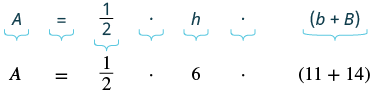Step 5. Solve the equation. $$\begin{split} A &= \dfrac{1}{2} \cdot 6(25) \\ A &= 3(25) \\ A &= 75\; square\; inches \end{split}$$ Step 6. Check: Is this answer reasonable?

If we draw a rectangle around the trapezoid that has the same big base B and a height h, its area should be greater than that of the trapezoid.

If we draw a rectangle inside the trapezoid that has the same little base b and a height h, its area should be smaller than that of the trapezoid.The area of the larger rectangle is 84 square inches and the area of the smaller rectangle is 66 square inches. So it makes sense that the area of the trapezoid is between 84 and 66 square inches

 Step 7. Answer the question. The area of the trapezoid is 75 square inches.
##### Exercise $$\PageIndex{27}$$:

The height of a trapezoid is 14 yards and the bases are 7 and 16 yards. What is the area?

161 sq. yd

##### Exercise $$\PageIndex{28}$$:

The height of a trapezoid is 18 centimeters and the bases are 17 and 8 centimeters. What is the area?

255 sq. cm

##### Example $$\PageIndex{15}$$:

Find the area of a trapezoid whose height is 5 feet and whose bases are 10.3 and 13.7 feet.

Solution

 Step 1. Read the problem. Draw the figure and label it with the given information.Step 2. Identify what you are looking for. the area of the trapezoid Step 3. Name. Choose a variable to represent it. Let A = the area Step 4.Translate. Write the appropriate formula. Substitute.Step 5. Solve the equation. $$\begin{split} A &= \dfrac{1}{2} \cdot 5(24) \\ A &= 12 \cdot 5 \\ A &= 60\; square\; feet \end{split}$$ Step 6. Check: Is this answer reasonable? The area of the trapezoid should be less than the area of a rectangle with base 13.7 and height 5, but more than the area of a rectangle with base 10.3 and height 5.Step 7. Answer the question. The area of the trapezoid is 60 square feet.
##### Exercise $$\PageIndex{29}$$:

The height of a trapezoid is 7 centimeters and the bases are 4.6 and 7.4 centimeters. What is the area?

42 sq. cm

##### Exercise $$\PageIndex{30}$$:

The height of a trapezoid is 9 meters and the bases are 6.2 and 7.8 meters. What is the area?

63 sq. m

##### Example $$\PageIndex{16}$$:

Vinny has a garden that is shaped like a trapezoid. The trapezoid has a height of 3.4 yards and the bases are 8.2 and 5.6 yards. How many square yards will be available to plant?

Solution

 Step 1. Read the problem. Draw the figure and label it with the given information.Step 2. Identify what you are looking for. the area of the trapezoid Step 3. Name. Choose a variable to represent it. Let A = the area Step 4.Translate. Write the appropriate formula. Substitute.Step 5. Solve the equation. $$\begin{split} A &= \dfrac{1}{2} \cdot (3.4)(13.8) \\ A &= 23.46\; square\; yards \end{split}$$ Step 6. Check: Is this answer reasonable? Yes. The area of the trapezoid is less than the area of a rectangle with a base of 8.2 yd and height 3.4 yd, but more than the area of a rectangle with base 5.6 yd and height 3.4 yd.Step 7. Answer the question. Vinny has 23.46 square yards in which he can plant.
##### Exercise $$\PageIndex{31}$$:

Lin wants to sod his lawn, which is shaped like a trapezoid. The bases are 10.8 yards and 6.7 yards, and the height is 4.6 yards. How many square yards of sod does he need?

40.25 sq. yd

##### Exercise $$\PageIndex{32}$$:

Kira wants cover his patio with concrete pavers. If the patio is shaped like a trapezoid whose bases are 18 feet and 14 feet and whose height is 15 feet, how many square feet of pavers will he need?

240 sq. ft

Perimeter of a Rectangle

Area of a Rectangle

Perimeter and Area Formulas

Area of a Triangle

Area of a Triangle with Fractions

Area of a Trapezoid

## Practice Makes Perfect

### Understand Linear, Square, and Cubic Measure

In the following exercises, determine whether you would measure each item using linear, square, or cubic units.

1. amount of water in a fish tank
2. length of dental floss
3. living area of an apartment
4. floor space of a bathroom tile
5. height of a doorway
6. capacity of a truck trailer

In the following exercises, find the (a) perimeter and (b) area of each figure. Assume each side of the square is 1 cm.

1.2.3.4.5.6.### Use the Properties of Rectangles

In the following exercises, find the (a) perimeter and (b) area of each rectangle.

1. The length of a rectangle is 85 feet and the width is 45 feet.
2. The length of a rectangle is 26 inches and the width is 58 inches.
3. A rectangular room is 15 feet wide by 14 feet long.
4. A driveway is in the shape of a rectangle 20 feet wide by 35 feet long.

In the following exercises, solve.

1. Find the length of a rectangle with perimeter 124 inches and width 38 inches.
2. Find the length of a rectangle with perimeter 20.2 yards and width of 7.8 yards.
3. Find the width of a rectangle with perimeter 92 meters and length 19 meters.
4. Find the width of a rectangle with perimeter 16.2 meters and length 3.2 meters.
5. The area of a rectangle is 414 square meters. The length is 18 meters. What is the width?
6. The area of a rectangle is 782 square centimeters. The width is 17 centimeters. What is the length?
7. The length of a rectangle is 9 inches more than the width. The perimeter is 46 inches. Find the length and the width.
8. The width of a rectangle is 8 inches more than the length. The perimeter is 52 inches. Find the length and the width.
9. The perimeter of a rectangle is 58 meters. The width of the rectangle is 5 meters less than the length. Find the length and the width of the rectangle.
10. The perimeter of a rectangle is 62 feet. The width is 7 feet less than the length. Find the length and the width.
11. The width of the rectangle is 0.7 meters less than the length. The perimeter of a rectangle is 52.6 meters. Find the dimensions of the rectangle.
12. The length of the rectangle is 1.1 meters less than the width. The perimeter of a rectangle is 49.4 meters. Find the dimensions of the rectangle.
13. The perimeter of a rectangle of 150 feet. The length of the rectangle is twice the width. Find the length and width of the rectangle.
14. The length of a rectangle is three times the width. The perimeter is 72 feet. Find the length and width of the rectangle.
15. The length of a rectangle is 3 meters less than twice the width. The perimeter is 36 meters. Find the length and width.
16. The length of a rectangle is 5 inches more than twice the width. The perimeter is 34 inches. Find the length and width.
17. The width of a rectangular window is 24 inches. The area is 624 square inches. What is the length?
18. The length of a rectangular poster is 28 inches. The area is 1316 square inches. What is the width?
19. The area of a rectangular roof is 2310 square meters. The length is 42 meters. What is the width?
20. The area of a rectangular tarp is 132 square feet. The width is 12 feet. What is the length?
21. The perimeter of a rectangular courtyard is 160 feet. The length is 10 feet more than the width. Find the length and the width.
22. The perimeter of a rectangular painting is 306 centimeters. The length is 17 centimeters more than the width. Find the length and the width.
23. The width of a rectangular window is 40 inches less than the height. The perimeter of the doorway is 224 inches. Find the length and the width.
24. The width of a rectangular playground is 7 meters less than the length. The perimeter of the playground is 46 meters. Find the length and the width.

### Use the Properties of Triangles

In the following exercises, solve using the properties of triangles.

1. Find the area of a triangle with base 12 inches and height 5 inches.
2. Find the area of a triangle with base 45 centimeters and height 30 centimeters.
3. Find the area of a triangle with base 8.3 meters and height 6.1 meters.
4. Find the area of a triangle with base 24.2 feet and height 20.5 feet.
5. A triangular flag has base of 1 foot and height of 1.5 feet. What is its area?
6. A triangular window has base of 8 feet and height of 6 feet. What is its area?
7. If a triangle has sides of 6 feet and 9 feet and the perimeter is 23 feet, how long is the third side?
8. If a triangle has sides of 14 centimeters and 18 centimeters and the perimeter is 49 centimeters, how long is the third side?
9. What is the base of a triangle with an area of 207 square inches and height of 18 inches?
10. What is the height of a triangle with an area of 893 square inches and base of 38 inches?
11. The perimeter of a triangular reflecting pool is 36 yards. The lengths of two sides are 10 yards and 15 yards. How long is the third side?
12. A triangular courtyard has perimeter of 120 meters. The lengths of two sides are 30 meters and 50 meters. How long is the third side?
13. An isosceles triangle has a base of 20 centimeters. If the perimeter is 76 centimeters, find the length of each of the other sides.
14. An isosceles triangle has a base of 25 inches. If the perimeter is 95 inches, find the length of each of the other sides.
15. Find the length of each side of an equilateral triangle with a perimeter of 51 yards.
16. Find the length of each side of an equilateral triangle with a perimeter of 54 meters.
17. The perimeter of an equilateral triangle is 18 meters. Find the length of each side.
18. The perimeter of an equilateral triangle is 42 miles. Find the length of each side.
19. The perimeter of an isosceles triangle is 42 feet. The length of the shortest side is 12 feet. Find the length of the other two sides.
20. The perimeter of an isosceles triangle is 83 inches. The length of the shortest side is 24 inches. Find the length of the other two sides.
21. A dish is in the shape of an equilateral triangle. Each side is 8 inches long. Find the perimeter.
22. A floor tile is in the shape of an equilateral triangle. Each side is 1.5 feet long. Find the perimeter.
23. A road sign in the shape of an isosceles triangle has a base of 36 inches. If the perimeter is 91 inches, find the length of each of the other sides.
24. A scarf in the shape of an isosceles triangle has a base of 0.75 meters. If the perimeter is 2 meters, find the length of each of the other sides.
25. The perimeter of a triangle is 39 feet. One side of the triangle is 1 foot longer than the second side. The third side is 2 feet longer than the second side. Find the length of each side.
26. The perimeter of a triangle is 35 feet. One side of the triangle is 5 feet longer than the second side. The third side is 3 feet longer than the second side. Find the length of each side.
27. One side of a triangle is twice the smallest side. The third side is 5 feet more than the shortest side. The perimeter is 17 feet. Find the lengths of all three sides.
28. One side of a triangle is three times the smallest side. The third side is 3 feet more than the shortest side. The perimeter is 13 feet. Find the lengths of all three sides.

### Use the Properties of Trapezoids

In the following exercises, solve using the properties of trapezoids.

1. The height of a trapezoid is 12 feet and the bases are 9 and 15 feet. What is the area?
2. The height of a trapezoid is 24 yards and the bases are 18 and 30 yards. What is the area?
3. Find the area of a trapezoid with a height of 51 meters and bases of 43 and 67 meters.
4. Find the area of a trapezoid with a height of 62 inches and bases of 58 and 75 inches.
5. The height of a trapezoid is 15 centimeters and the bases are 12.5 and 18.3 centimeters. What is the area?
6. The height of a trapezoid is 48 feet and the bases are 38.6 and 60.2 feet. What is the area?
7. Find the area of a trapezoid with a height of 4.2 meters and bases of 8.1 and 5.5 meters.
8. Find the area of a trapezoid with a height of 32.5 centimeters and bases of 54.6 and 41.4 centimeters.
9. Laurel is making a banner shaped like a trapezoid. The height of the banner is 3 feet and the bases are 4 and 5 feet. What is the area of the banner?
10. Niko wants to tile the floor of his bathroom. The floor is shaped like a trapezoid with width 5 feet and lengths 5 feet and 8 feet. What is the area of the floor?
11. Theresa needs a new top for her kitchen counter. The counter is shaped like a trapezoid with width 18.5 inches and lengths 62 and 50 inches. What is the area of the counter?
12. Elena is knitting a scarf. The scarf will be shaped like a trapezoid with width 8 inches and lengths 48.2 inches and 56.2 inches. What is the area of the scarf?

## Everyday Math

1. Fence Jose just removed the children’s playset from his back yard to make room for a rectangular garden. He wants to put a fence around the garden to keep out the dog. He has a 50 foot roll of fence in his garage that he plans to use. To fit in the backyard, the width of the garden must be 10 feet. How long can he make the other side if he wants to use the entire roll of fence?
2. Gardening Lupita wants to fence in her tomato garden. The garden is rectangular and the length is twice the width. It will take 48 feet of fencing to enclose the garden. Find the length and width of her garden.
3. Fence Christa wants to put a fence around her triangular flowerbed. The sides of the flowerbed are 6 feet, 8 feet, and 10 feet. The fence costs $10 per foot. How much will it cost for Christa to fence in her flowerbed? 4. Painting Caleb wants to paint one wall of his attic. The wall is shaped like a trapezoid with height 8 feet and bases 20 feet and 12 feet. The cost of the painting one square foot of wall is about$0.05. About how much will it cost for Caleb to paint the attic wall?## Writing Exercises

1. If you need to put tile on your kitchen floor, do you need to know the perimeter or the area of the kitchen? Explain your reasoning.
2. If you need to put a fence around your backyard, do you need to know the perimeter or the area of the backyard? Explain your reasoning.
3. Look at the two figures. (a) Which figure looks like it has the larger area? Which looks like it has the larger perimeter? (b) Now calculate the area and perimeter of each figure. Which has the larger area? Which has the larger perimeter?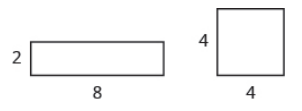1. The length of a rectangle is 5 feet more than the width. The area is 50 square feet. Find the length and the width. (a) Write the equation you would use to solve the problem. (b) Why can’t you solve this equation with the methods you learned in the previous chapter?

## Self Check

(a) After completing the exercises, use this checklist to evaluate your mastery of the objectives of this section.(b) On a scale of 1–10, how would you rate your mastery of this section in light of your responses on the checklist? How can you improve this?